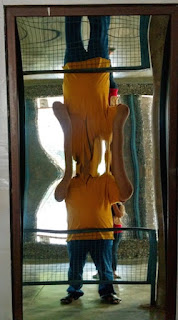## Reflection of Light By Plane and Spherical MirrorsLaughing Mirrors use combination of Spherical Mirrors and Plane Mirrors. Is this image real or virtual?

### Very Short Question Answers (Based on NCERT Chapter)

Q1: What is the position of the object, when we get a diminished image with a concave mirror?

Answer: Beyond C (centre of curvature)

Q2: What are Marginal rays?

Answer: The rays which strike the mirror surface near the periphery are called marginal rays.

Q3: What type of rear mirrors are used by drivers in automobiles?

Q4: What is the relation between the radius of curvature (R) and focal length (f) of a concave mirror?

Answer: R = 2f

Q5: What is a focal length in case of spherical mirrors?

Answer: It is the distance between focal length and focus.

Q6: An object is moving with a speed v towards a plane mirror, what is the speed of the image?

Q7: What term we use to define the geometric centre of concave or convex mirror?

Q8:  What type of images are formed by a concave mirror?

Answer: Both virtual and real.

Q9: What types of mirrors are used in headlights of vehicles?

Q10: According to sign conventions, the Radius of curvature of concave mirror is considered as +ve or -ve?

Q11: What type of images cannot be taken on screen?

Q12: Can camera capture virtual images?

Q12: If value of magnification is 1, what will be relation between image and the object?

Answer: Size of image equals to size of the object.

Q13: Why does image appear thin or bulging in spherical mirrors?

Answer: In spherical mirrors, light ray converge or diverge upon reflection, hence appearing thin or bulged.

Q14: What is the magnification of a plane mirror?

Q15: What type of mirrors are used by dentists or ENT specialists?

Q16: If magnification m > 1, is the image formed enlarged or reduced?

Q17: What types of mirrors are used in saloons?

Q18:  If magnification m < 0, is the image formed erect or inverted?

Q19: Where will the images of stars or the moon be formed for a concave mirror?

Answer: At focus of mirror. Image will be point sized.

Q20: Find the focal length of a convex mirror whose radius of curvature is 32 cm?

Answer:  R = 32cm. f = R/2 = 32/2 = 16 cm

Q21: Name a mirror that can give an erect and enlarged image of an object.

Q22: No matter how far you stand from a mirror, your image appears erect. The mirror is likely to be _____?

Answer: either plane or convex mirror.

Q23: How many images do we see between two parallel mirrors?

Q24: If the image is not there where light goes after reflection, such image is called as?

Q25: Will the value of magnification of a rear mirror used in car is positive or negative?

Answer: Positive (+m < 1) because image needs to be erect.

Q26: What is the focal length of a plane mirror? (zero or infinite)

Q27: A light ray parallel to principal axis falls on a concave mirror and bends towards focus. Will angle of incidence be equal to angle of reflection?

Q28 (HOTS): Can a convex mirror or plane mirror form a real image?

Answer: Usually convex mirror or plane mirror forms virtual images. But it can form a real image, if the object is virtual, i.e., if the light rays converging at a point behind a plane mirror (or a convex mirror) are reflected to a point on a screen placed in front of the mirror, then a real image will be formed. eg Throw a torch at the plane mirror.